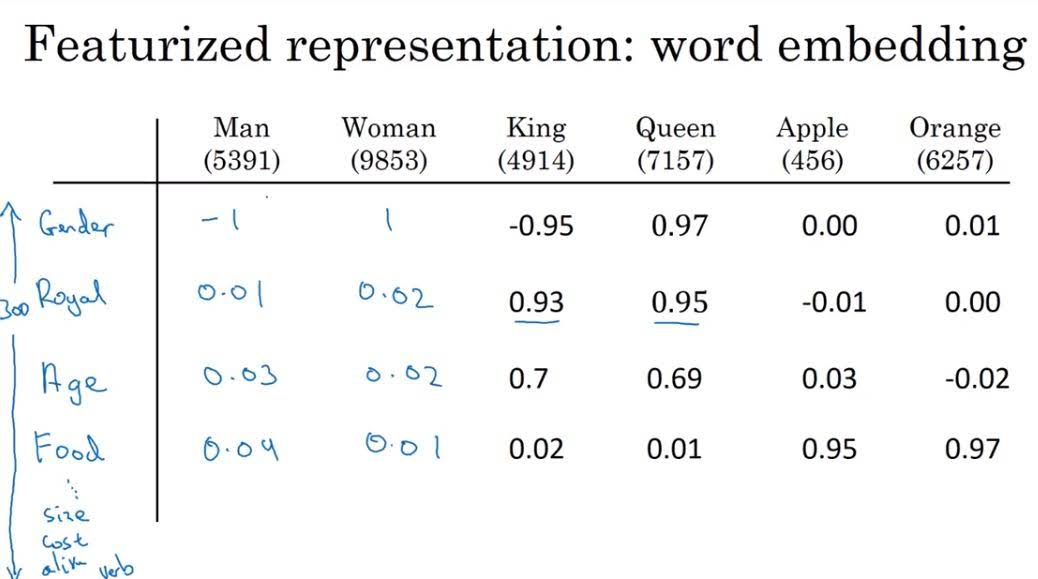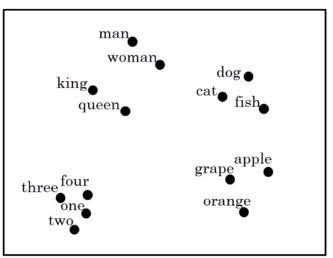Categories:

Updated:

# What is Word Embedding?

Until now, we’ve represented word with one-hot vector that tells number of index the word appears in vocabulary. However, this kind of word representation is arbitrary; it doesn’t capture relationships like man-woman, king-queen, orange-apple, etc.

Word embedding is a vector representation of a word in a ‘meaningful’ feature space. It is like face encoding, except that it only can encode words in a certain vocabulary.

For example, we can embed word with features like ‘gender’, ‘age’, ‘food’, etc.Then, the distance between apple and orange would be closer than distance between apple and man. So our model can learn that if ‘juice’ fits well into the ‘He drinks apple ()’, ‘juice’ also fits well into the ‘He drinks orange ()’.

In practice, we don’t set these features ourselves because doing so is a waste of time and also an arbitrary thus inefficient representation. Instead, word embeddings are ‘learned’ from a large text corpus.

Below is a 2-dimensional t-SNE visualization of word embeddings. You can find out similar words belong to the same cluster.# Using Word Embeddings

Representing words is the first step in NLP tasks. So using the pre-trained word embeddings as word representations itself will enhance the model’s performance.

Especially, word embeddings are usefull when you only have small dataset for specific NLP task. You can learn word embeddings from general large text corpus yourself or download pre-trained word embeddings and use them to train RNN for your task. This is another type of transfer learning, since the information learned from large corpus is transfered to a specific task.

# Learning Analogies

You can understand the key concept of word embeddings by understanding that word embeddings can be used to learn analogies. For example, word embeddings can be used to answer to the question like ‘If man is to woman, then king is to what?’.

We can answer this question by doing this;

where $w$ is the word we want to find and $e_x$ is an embedding of word $x$.

We usually use cosine similarity for similarity function;

Hopefully, with a well trained word embedding, our algorithm will say that $w$ is a word ‘queen’.

# Embedding Matrix

What you mean by ‘learning word embeddings’ actually means learning ‘embedding matrix’.

If you want to embed $n$ words including <UNK> and <EOS> with $k$ features, your embedding matrix’s shape would be $k\times n$, where each column represents embedding vector of each word.

To get embedding vector of an $i$th word, you calculate $e_i = Eo_i$ where $o_i$ is a one-hot vector representation of word $i$.

Categories: